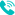0553-5896615

# 交流伺服电机工作原理_调速控制_优缺点

IC先生 IC先生 762 2022-12-14 14:34:35

### 基本概念### 结构特点### 工作原理### 传递函数• Tm=电机产生的扭矩。
• Tl=负载产生的扭矩或负载扭矩。
• 'θ'=角位移。
• 'ω'=dθ/dt=角速度。
• 'J'=负载的转动惯量。
• 'B'=负载的阻尼器。

• 'K1'是控制相电压与转矩特性的斜率。
• 'K2'是速度转矩特性的斜率。

Tl = TJ + TB = J d^2θ/dt^2 + B dθ/dt^2 + B —–(2)

K1ec- K2 dθ/dt = J d^2θ/dt^2 + B dθ/dt^2 + B

K1Ec(s) – K2 S θ(S) = JS^2θ (S) + BS θ(S)

K1Ec(s) = JS^2θ(S) + BSθ(S)+ K2S θ(S)

K1Ec(s) = θ(S)[JS^2 + BS + K2S]

TF = θ (S)Ec(s) = K1/ JS^2 + BS + K2S= K1/ S [B + JS + K2]= K1/ S [B + K2 + JS]= K1/ S (B + K2) [1 + (J/ B + K2) *S]

TF = θ (S)Ec(s) = K1/(B + K2) / S[1 + (J/ B + K2) *S]

TF = Km / S[1 + (J/ B + K2) *S] => Km / S(1 + STm)] = θ (S)Ec(s)

TF = Km / S(1 + STm)] = θ (S)Ec(s)

### 调速控制方法

1. 位置控制方法用于确定整个外部输入频率信号的旋转速度的大小。旋转角度由脉冲数决定。伺服电机的位置和速度可以通过通信直接分配。由于该方法的位置可以对位置和速度进行极其严格的控制，因此通常在定位应用中使用。
2. 在转矩控制方式中，伺服电机的输出转矩由地址处的模拟量输入设定。它可以通过简单地实时改变模拟来改变扭矩。此外，它还可以通过通信改变相对地址处的值。
3. 在速度控制模式下，电机速度可以通过模拟量输入和脉冲来控制。如果有精度要求并且不关心那么大的扭矩，那么速度模式更好。

### 主要特性### 优缺点

• 速度控制特性良好。
• 产生的热量较少。
• 提供高效率、更大的单位重量扭矩、可靠性和更低的射频噪声。
• 需要更少的维护。
• 在没有换向器的情况下，它们的预期寿命更长。
• 能够处理工业机械中更高的电流浪涌。
• 在高速下，它们提供更恒定的扭矩。
• 都是高度可靠的。
• 提供高速性能。
• 非常适合不稳定的负载应用。

• 控制难度较大。
• 可能因持续过载而损坏。
• 齿轮箱经常需要高速传输动力。

### 主要应用

• 适用于位置调节很重要的地方，通常用于半导体设备、机器人、飞机和机床。
• 用于在伺服机构上运行的仪器，如计算机和位置控制设备。
• 用于机床、机器人机械和跟踪系统。
• 用于大多数常见的机器和电器，如热水器、烤箱、泵、越野车、花园设备等。

### 总结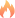热点资讯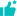推荐商品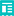猜你想看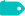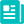热点资讯